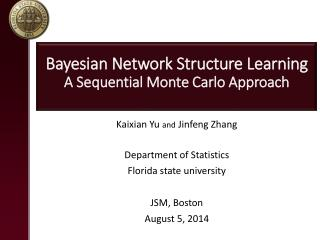# Bayesian Network Structure Learning A Sequential Monte Carlo Approach - PowerPoint PPT PresentationDownload PresentationBayesian Network Structure Learning A Sequential Monte Carlo Approach

Bayesian Network Structure Learning A Sequential Monte Carlo ApproachDownload Presentation## Bayesian Network Structure Learning A Sequential Monte Carlo Approach

- - - - - - - - - - - - - - - - - - - - - - - - - - - E N D - - - - - - - - - - - - - - - - - - - - - - - - - - -
##### Presentation Transcript

1. Bayesian Network Structure Learning A Sequential Monte Carlo Approach Kaixian Yu andJinfeng Zhang Department of Statistics Florida state university JSM, Boston August 5, 2014

2. What is Bayesian Network? • A Bayesian network (BN), , is a graphical representation of joint probability distribution. • The structure of BN is a Directed Acyclic Graph (DAG) encodes the conditional dependencies among the random variables (nodes in graph). • BN has various applications in Biology, text mining, and etc.

3. Structure Learning and challenges • The goal of structure learning is to reveal all conditional dependencies and independencies (CDIs) of variables. • Challenge: Ultra-High dimension structure space, the dimension is where is number of nodes. Example: The cardinality of DAG space with 8 nodes is approximately • Basic learning strategies: constraint, score, and hybrid. • Constraint based: identify CDIs by conditional dependency test • Score based: optimize a score function measuring the fitness of a network. • Hybrid (two stages): a mix of constraint and score based method • Constraint based: identify CDIs by conditional dependency test • Score based: optimize a score function measures the fitness of a network. • Hybrid (two stages): a mix of constraint and score based method

4. More on Hybrid methods • Stage one: identify the undirected edges (connections) by conditional dependency test. • Stage two: optimize a specified score function based on the undirected graph learned from stage one. • We designed a two-stage hybrid method: Double-Filtering-Sequential-Monte-Carlo (DFSMC). • First stage: We concentrate on the ability of identifying as many true connections as possible while propose fewer connections. • Second stage: SMC is adopted to optimize the BIC score. *Issue: Missed edge in stage one will never be reclaimed. • Stage one: identify the undirected edges (connections) by conditional dependency test. • Stage two: optimize a specified score function based on the undirected graph learned from stage one.

5. Learning Basics • Equivalent BNs: and is called equivalent when they represents the same CDIs. Example: and is equivalent. • Score equivalence: A given score function is said to be score equivalence if for two equivalent BNs and , always hold. Example: Bayesian Information Criterion (BIC).

6. Double Filtering Algorithm: Theorem 1: • First filtering: conduct dependency test for each pair of nodes and ; update the dependent set of , . • Second filtering : Update for all = 1,2,…,N, by conditional dependency test conditioning on each node in . When observation size contains all true neighbors of

7. SMC search Algorithm: When there exists at least one connected triplet, sample a triplet from the candidates with least outside connections; Sample a configuration of the triplets according to their BICs; Repeat I and II until no connected triplet left. Sample a connected pair; Sample a direction (configuration) according to the BICs; Repeat IV and V until no connections left.

8. Simulation study Benchmark networks: Data generating • For each network 3 sizes of observations are considered (2k, 5k, 10k). • For each size of observations 20 independent datasets are generated.

9. Result: edge detection (recall) DF Mmpc Shp cl

10. Result: edge detection (f score) DF Mmpc Shp cl

11. Result: BIC DF Mmhc true

12. Summary • We Proposed a two stage BN structure learning method DFSMC • First stage: detect as many true edges and reasonable trade-off • Requires less observations to conduct test • Second stage: SMC, diversity and run time trade off. • Parallelizable, short run time for each SMC sample.

13. Future work • Compare to more methods for edge detection and learned network. • Scale up our method to larger networks and more observations. • Add one more stage trying to recover the edges missed from stage one.

14. Thank you! Comments and Questions?

15. Discussion: temperature